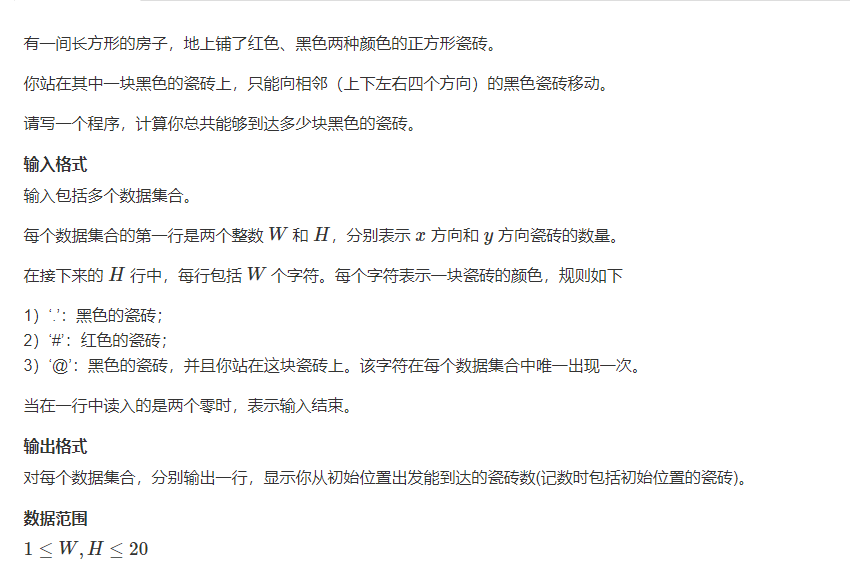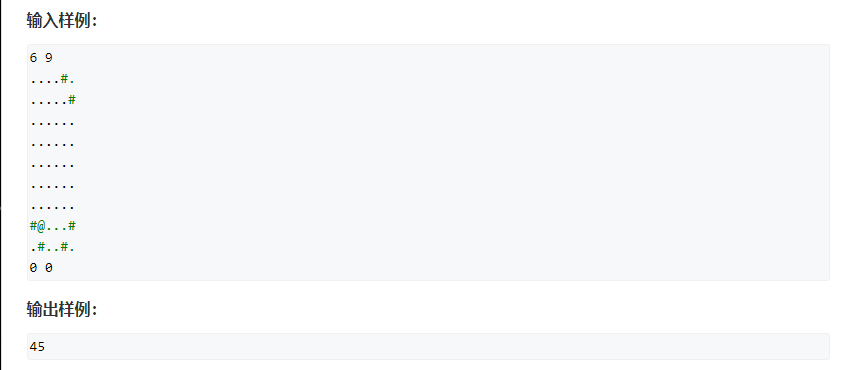# 题目

• 输入包括多个数据集合。
• 每个数据集合的第一行是两个整数 W 和 H，分别表示 x 方向和 y 方向瓷砖的数量。
• 在接下来的 H 行中，每行包括 W 个字符。每个字符表示一块瓷砖的颜色，规则如下
• 1）‘.’：黑色的瓷砖；
• 2）‘#’：红色的瓷砖；
• 3）‘@’：黑色的瓷砖，并且你站在这块瓷砖上。该字符在每个数据集合中唯一出现一次。
• 当在一行中读入的是两个零时，表示输入结束。

• 对每个数据集合，分别输出一行，显示你从初始位置出发能到达的瓷砖数(记数时包括初始位置的瓷砖)。

• 1 ≤ W , H ≤ 20 1≤W,H≤20
输入样例：
6 9
....#.
.....#
......
......
......
......
......
#@...#
.#..#.
0 0

45# 详细题解

#### 写法1 O ( n ∗ m ) O(n*m)

bfs:

#include <iostream>
#include <cstring>
#include <algorithm>
#include <queue>

#define x first
#define y second

using namespace std;

typedef pair<int, int> PII;
const int N = 25;

int n, m;
char f[N][N];

int bfs(int sx, int sy)
{
queue<PII> q;
q.push({sx, sy});
f[sx][sy] = '#';
int ans = 0;

int dx[] = {-1, 0, 1, 0}, dy[] = {0, 1, 0, -1};

while (q.size())
{
auto t = q.front();
q.pop();
++ ans;
for (int i = 0; i < 4; ++ i )
{
int x = t.x + dx[i], y = t.y + dy[i];
if(x < 0 || x >= n || y < 0 || y >= m || f[x][y] != '.') continue;
f[x][y] = '#';
q.push({x, y});
}
}
return ans;
}

int main()
{
while(cin >> m >> n, n || m)
{
for (int i = 0; i < n; ++ i ) cin >> f[i];
int x, y;
for (int i = 0; i < n; ++ i )
for (int j = 0; j < m; ++ j )
if (f[i][j] == '@')
{
x = i; y = j;
}
cout << bfs(x, y) << endl;
}

return 0;
}#### 推导证明#### 写法2 O ( n ∗ m ) O(n*m)

dfs:

#include <iostream>

using namespace std;

const int N = 25;

int n, m;
char f[N][N];
int dx[] = {-1, 0, 1, 0}, dy[] = {0, 1, 0, -1};

int dfs(int sx, int sy)
{
int ans = 1;
f[sx][sy] = '#';
for (int i = 0; i < 4; ++ i )
{
int x = sx + dx[i], y = sy + dy[i];
if (x >= 0 && x < n && y >=0 && y < m && f[x][y] == '.')
{
ans += dfs(x, y);
}
}

return ans;
}

int main()
{
while(cin >> m >> n, n || m)
{
for (int i = 0; i < n; ++ i ) cin >> f[i];
int x, y;
for (int i = 0; i < n; ++ i )
for (int j = 0; j < m; ++ j )
if (f[i][j] == '@')
{
x = i; y = j;
}
cout << dfs(x, y) << endl;
}

return 0;
}#### 推导证明# 总结04-1745302-101608
01-1983
03-282977
03-05353
03-0213
10-17443
09-2228
01-1259
01-2655
01-2122
01-28245
01-1230
02-08105
10-011250
08-26210
09-041130